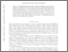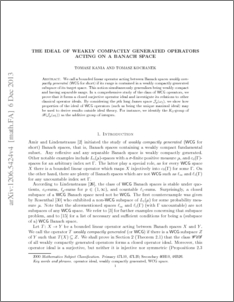# The ideal of weakly compactly generated operators acting on a Banach space

Kania, Tomasz and Kochanek, Tomasz (2014) The ideal of weakly compactly generated operators acting on a Banach space. Journal of Operator Theory, 71 (2). pp. 455-477. ISSN 0379-4024Preview
PDF (1206.5424)
1206.5424.pdf - Submitted Version

## Abstract

We call a bounded linear operator acting between Banach spaces \textit{weakly compactly generated} ($\mathsf{WCG}$ for short) if its range is contained in a~weakly compactly generated subspace of its target space. This notion simultaneously generalises being weakly compact and having separable range. In a comprehensive study of the class of $\mathsf{WCG}$ operators, we prove that it forms a~closed surjective operator ideal and investigate its relations to other classical operator ideals. By considering the $p$th long James space $\J_p(\om_1)$, we show how properties of the ideal of $\mathsf{WCG}$ operators (such as being the unique maximal ideal) may be used to derive results outside ideal theory. For instance, we identify the $K_0$-group of $\B(\J_p(\om_1))$ as the additive group of integers and prove automatic continuity of homomorphisms from this Banach algebra.

Item Type:
Journal Article
Journal or Publication Title:
Journal of Operator Theory
Uncontrolled Keywords:
/dk/atira/pure/subjectarea/asjc/2600/2602
Subjects:
Departments:
ID Code:
73762
Deposited By:
Deposited On:
18 Jun 2015 05:44
Refereed?:
Yes
Published?:
Published